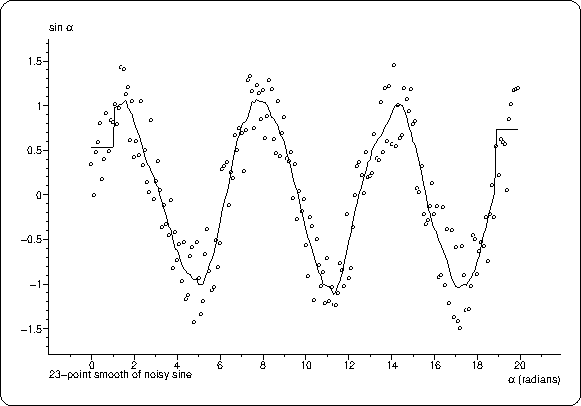# plsmooth

## performs a k-point smoothing on an array

parametertypeunitsdescription
arrayfloat*uuarray to be smoothed
nint--length of array
kint--number of points of the smooth (must be odd)
returns:void

## Description

plsmooth applies a k-point (k must be odd and < n) quartic-cubic smooth to the n values in array. The first and last k/2 points in a are unaffected.

see c.g.enke, t.a.nieman, anal. chem. vol 48, page 705a

## Examples

```/* __________ plsmooth __________
plotting a noise modulated sine with and without smoothing
features: use of plaxfit, plpline, plformat
smoothing
*/
#include <stdlib.h>
#include <math.h>
#include <simplot.h>
#define NPOINTS 200
#define RANGE 23
int main() {
float x[NPOINTS],y[NPOINTS];
int i;
plinit(PS,"plsmooth",A4,50,50,"","");

for (i=0;i<NPOINTS;i++)     // loop generates the noise-modulated sine data
{ x[i]=i/10.;
y[i]=sin(x[i])+(float)rand()/RAND_MAX-.5;
}
// fits axes to the data
plotm(0,0,UP);
plformat(.5,-.5,"\n%d-point smooth of noisy sine",RANGE);
// report smoothing range
plset(HEIGHT,1);                // make symbols small giving dots
plpline(x,y,NPOINTS,"O",UP);    /* plot unsmoothed data.
mark with O's, no connecting line */
if (plget(PLOTTER)!=PS)
plcolor(Red);
plsmooth(y,NPOINTS,RANGE);      // smooth the data
plpline(x,y,NPOINTS,"",DOWN);   // and plot again. no marking symbols
plframe(5,3);
plend();
exit(0);
}
```• Home